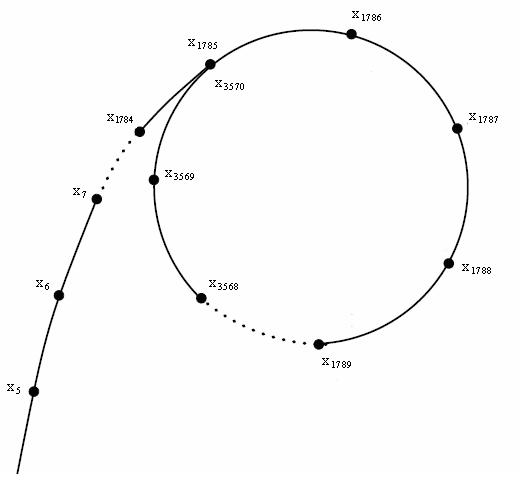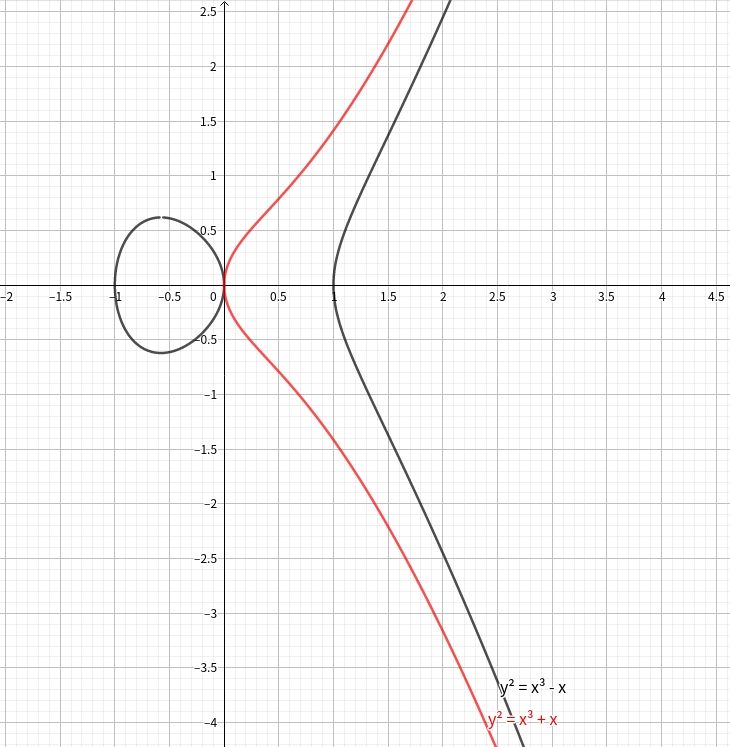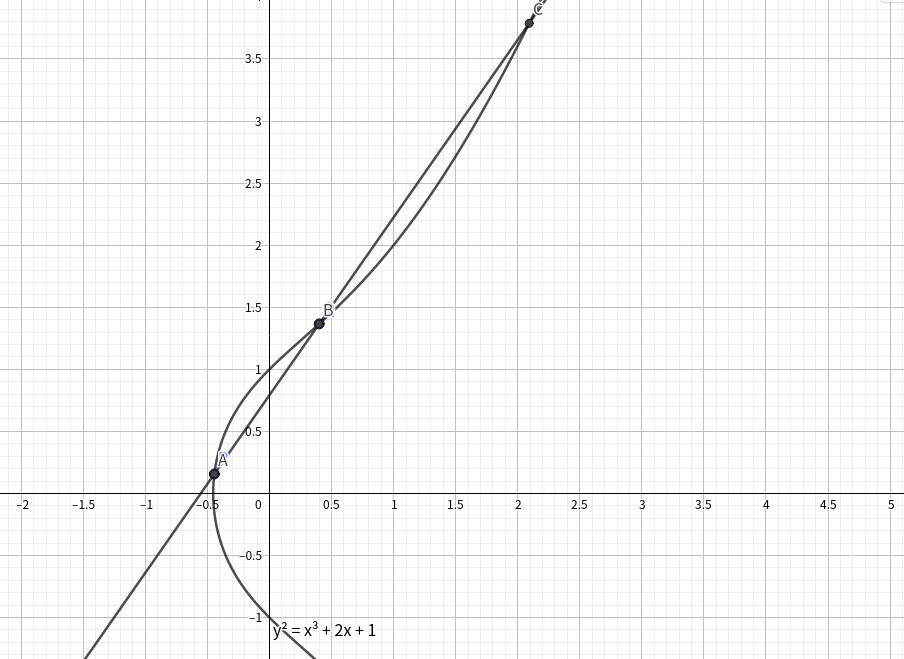博客

$\mathbb Z$ is difficult polynomials are easy

2021-03-21 20:21:38 By computerkiller

$\mathbb Z$ is difficult polynomials are easy

from this

General

$\mathbb Z$ 和 polynomial 上有很多相似问题 比如 $\mathbb Z$ 的 质因数分解 可以 对应到 polynomial 的 因式分解

Example

Fermat's Last Theorem

$a^n+b^n=c^n$ 当 $n \geq 3$ 时没有正整数解

$f,g,h\in \mathbb C[x]$ 且 $\gcd(f,g,h)=1$ 求证 当 $n\geq 3$ 时 $f^n+g^n=h^n\to f,g,h \in \mathbb C$

ABC-conjecture

ABC 猜想 是:

Lemma1: $$\deg f \leq \deg\gcd(f,f^{\prime})+\operatorname{N}(f)$$ 证明的话 可以假设 $(x-c)^n\mid f$ 那么 $f=(x-c)^n \hat f$ 那么 $f^{\prime}=(x-c)^n \hat f^{\prime}+n(x-c)^{n-1}\hat f$

$f,g,h\in \mathbb C[x]$ 且 $\gcd(f,g,h)=1$ 求证 当 $\frac{1}{p}+\frac{1}{q}+\frac{1}{r}\leq 1$ 时 $f^p+g^q=h^r\to f,g,h \in \mathbb C$

Conclusion

Be Happy If You Find A Locally Nilpotent Derivation on your ring

如何优雅地进行质因数分解

2020-12-10 20:59:51 By computerkiller

如何优雅地进行质因数分解

Miller–Rabin primality test

Proof: $$\text{我们假设}x\text{是}1\text{在模p意义下的一个非平凡平方根,p是奇质数}\\ x^2\equiv 1\ (mod\ p)\\ (x-1)(x+1)\equiv0\ (mod\ p)\\ \text{即}p|(x-1)(x+1)\\ \text{根据欧几里得引理得到:}p|x-1\text{或}p|x+1\\ \text{此时}x\text{必然是平凡平方根,与题设矛盾,所以引理成立}\\$$ 接下来解释一下算法的原理:

int mul(int a,int b,int mod)
{
int tmp = a * b - (int) ((long double) a * b / mod + 0.5) * mod;
return tmp < 0 ? tmp + mod : tmp;
}

int ksm(int a,int b,int mod = mod)
{
int res = 1;
while (b)
{
if (b & 1) res = mul(res,a,mod);
a = mul(a,a,mod);
b >>= 1;
}
return res;
}

int check(int a,int n)
{
int b = (n - 1),k = 0;
while (!(b & 1))
{
b >>= 1;
k++;
}
int res = ksm(a,b,n);
if (res == 1 || res == n - 1) return 0;
while (k--)
{
res = mul(res,res,n);
if (res == n - 1) return 0;
}
return 1;
}

int Miller_Robin(int n)
{
if (n == 2 || n == 3 || n == 5 || n == 7 || n == 11 || n == 13 || n == 17 || n == 19) return 1;
if (n == 23 || n == 29 || n == 31 || n == 37 || n == 41) return 1;
if (n == 46856248255981ll) return 0;
if (n < 2 || !(n & 1)) return 0;
if (!(n % 3 && n % 5 && n % 7 && n % 11 && n % 13 && n % 17 && n % 19)) return 0;
if (!(n % 23) || !(n % 29) || !(n % 31) || !(n % 37) || !(n % 41)) return 0;
if (check(2,n)) return 0;
if (check(3,n)) return 0;
if (check(5,n)) return 0;
if (check(7,n)) return 0;
if (check(11,n)) return 0;
if (check(13,n)) return 0;
if (check(17,n)) return 0;
if (check(19,n)) return 0;
if (check(23,n)) return 0;
if (check(29,n)) return 0;
if (check(31,n)) return 0;
if (check(37,n)) return 0;
if (check(41,n)) return 0;
return 1;
}

Trial division

map<int,int> fac;
for (int i = 2; i * i <= n; i++)
{
while (!(n % i))
{
n /= i;
fac[i]++;
}
}
if (n != 1) fac[n]++;

Wheel factorization

map<int,int> fac;
while (!(n % 2))
{
n /= 2;
fac++;
}
for (int i = 3; i * i <= n; i += 2)
{
while (!(n % i))
{
n /= i;
fac[i]++;
}
}
if (n != 1) fac[n]++;

Precomputed primes

int pri[N],cnt = 0;
bool vis[N];
map<int,int> fac;
for (int i = 2; i < N; i++)
{
if (!vis[i]) pri[++cnt] = i;
for (int j = 1; j <= cnt && i * pri[j] < N; j++)
{
vis[i * pri[j]] = 1;
if (!(i % pri[j])) break;
}
}
for (int i = 1; i <= cnt && pri[i] * pri[i] <= n; i++)
{
while (!(n % pri[i])) mp[pri[i]]++,n /= pri[j];
}
for (int d = pri[cnt] + 1,i = 0; d * d <= n; d += pri[++i])
{
while (!(n % d))
{
n /= d;
fac[d]++;
}
if (i == cnt) i = 0;
}
if (n != 1) fac[n]++;

Fermat's factorization method

int fermat(int n) {
int a = ceil(sqrt(n));
int b2 = a * a - n;
int b = round(sqrt(b2));
while (b * b != b2) {
a = a + 1;
b2 = a * a - n;
b = round(sqrt(b2));
}
return a - b;
}

Pollard's rho algorithm

Linux自带的factor中所使用的算法 期望复杂度在$O(n^{\frac{1}{4}})$ 算法导论上说是$O(\sqrt{p})$ $p$是$n$的最小质因子

void fac(int n)
{
if (isprime(n)) return ;
int p = find(n);
fac(p);
fac(n / p);
}Floyd's cycle-finding algorithm

Floyd的这个算法据说是民间算法/? 不管

Brent's algorithm

int Pollard_Rho(int x)
{
int s = 0,t = 0;
int c = (int) rand() % (x - 1) + 1;
int step = 0,goal = 1;
int val = 1;
for (goal = 1;; goal <<= 1,s = t,val = 1)
{
for (step = 1; step <= goal; ++step)
{
t = f(t,c,x);
val = mul(val,abs(t - s),x);
if ((step % 127) == 0)
{
int d = gcd(val,x);
if (d > 1) return d;
}
}
int d = gcd(val,x);
if (d > 1) return d;
}
}

void fac(int x,int num)
{
if (x < 2) return ;
if (Miller_Robin(x))
{
maxx = max(maxx,x);
return ;
}
int p = x;
while (p >= x) p = Pollard_Rho(x);
int tmp = 0;
while ((x % p) == 0) x /= p,tmp += num;
fac(x,num);
fac(p,tmp);
}

Algebraic-group factorisation algorithm

Pollard's p - 1 algorithm

1. 选定一个$B$ 计算$x$ 选定一个$a$
2. 计算$g = gcd(a^x-1,n)$
3. 如果 $g = 1$ 那么调大$B$ 返回步骤 $2$ 或者分解失败
4. 如果 $g = n$ 那么减小$B$返回步骤 $2$ 或者分解失败​
5. 得到一个非平凡因子$g$

Lenstra elliptic-curve factorization

椭圆曲线1. 选取一个椭圆曲线$E$和上面在群中的一个元素$P=(x,y)$
2. 选定一个值$B$ 这和Pollard's p - 1很类似 对于$10^{30}$位左右的数 我们一般选取$700$
3. 计算$\left(\displaystyle\prod\limits_{p\in prime,p 4. 在加法求逆的过程中 寻找点$O$复杂度分析类似于Pollard's p - 1 我们当$|(\mathcal Z / n,\mathcal Z)|$是B-smooth的时候停下 复杂度是:$O(B\log^2n)$终止概率一样是$B=p^\frac{1}{a},P=a^{-a}$所以$T(n)=e^{(\sqrt{2}+o(1))\sqrt{\log p\log\log p}}p$是$n$最大的因子 到此 我们可以解决FACT2 浅谈树上的两个小trick 2020-09-25 14:35:22 By computerkiller 这份Blog将会包括两部分 长链剖分和dsu on tree 我们先讲一些前置知识$\color{red}{轻重链剖分}$吧 我们一般认为的树链剖分默认为轻重链剖分 主要的思想在于让重链上的dfn序连续 从而将树上的问题转化成几个区间的问题 考虑如何做到这件事情: 在第一次dfs的时候 我们便要去处理出来重儿子 方便第二次去进行剖分 这件事情很$naive$就是在dfs的过程中去记录下重儿子的序号 void dfs1(int u,int f) { dep[u] = dep[f] + 1; fa[u] = f; son[u] = -1; siz[u] = 1; for (int i = head[u]; i != -1; i = nxt[i]) { int v = pnt[i]; if (v == f) continue; dfs1(v); siz[u] += siz[v]; if (son[u] == -1 || siz[v] > siz[son[u]]) son[u] = v; } } 在第二次dfs的时候 优先遍历重儿子 再遍历轻儿子 这样就可以保证重链上的dfn序连续 void dfs2(int u,int tp) { top[u] = tp; dfn[u] = ++cnt; rnk[cnt] = u; if (son[u] == -1) return ; dfs2(son[u],tp); for (int i = head[u]; i != -1; i = nxt[i]) { int v = pnt[i]; if (v == fa[u] || v == son[u]) continue; dfs2(v,v); } } 很明显这样剖分完了之后 我们做到了重链上dfn序连续 并且注意到 我们这样剖分完会产生$logn$条链 那么我们来看一下树剖之后的小常数求lca int query(int x,int y) { while (top[x] != top[y]) { if (dep[top[x]] < dep[top[y]]) swap(x,y); x = fa[top[x]]; } if (dep[x] > dep[y]) swap(x,y); return x; } 这样子寻找lca的常数很小倍增留下了泪水 dsu on tree 到这里 我们花了60+行解释了树剖 终于引出第一个主题 dsu on tree dsu on tree基于的是树剖的思想 让重儿子的贡献直接继承到父亲上 而轻儿子的考虑暴力合并 无论你是logn - logs的推式子大师 还是每次操作后规模减半的毛估估大师 都能发现这个的复杂度是nlogn的 在处理子树问题时 吊打了树上莫队 我们看一下这样一个代码 我们以CF600E为例题 分析一下算法的流程: void dfs(int u) { for (int i = head[u]; i != -1; i = nxt[i]) { int v = pnt[i]; if (v == fa[u] || v == son[u]) continue; dfs(v); } if (son[u] != -1) dfs(son[u]); for (int i = head[u]; i != -1; i = nxt[i]) { int v = pnt[i]; if (v == fa[u] || v == son[u]) continue; dsu(v,1); } tmp[t[col[u]]] -= col[u]; t[col[u]]++; tmp[t[col[u]]] += col[u]; if (tmp[maxx + 1]) maxx++; if (!tmp[maxx]) maxx--; ans[u] += tmp[maxx]; if (u == top[u]) dsu(u,-1); } 这个是在树链剖分之后的一个计算答案的dfs 其中的top fa son这些数组和在树链剖分中的同义 在这个过程中 不难发现的是 我们后遍历了重儿子 这是和树剖不同的地方 原因很简单 我们遍历轻儿子是为了计算轻儿子的答案 而我们的重儿子是直接继承的 所以先遍历的话会影响到轻儿子的答案的计算 于是我们有了这样的一个算法流程: 1.计算轻儿子的答案 2.遍历重儿子继承重儿子的答案 3.将轻儿子的贡献暴力计算 4.加上自己的贡献 计算自己的答案 5.如果自己是某条重链的头 那么自己必然是某个结点的轻儿子了 此时我们需要消除我们这棵子树的贡献 上面代码中的dsu函数是对于子树遍历的 我给出我的计算方式: void dsu(int u,int k) { tmp[t[col[u]]] -= col[u]; t[col[u]] += k; tmp[t[col[u]]] += col[u]; if (tmp[maxx + 1]) maxx++; if (!tmp[maxx]) maxx--; for (int i = head[u]; i != -1; i = nxt[i]) { int v = pnt[i]; if (v == fa[u]) continue; dsu(v,k); } } 这样dsu on tree的算法部分就讲完了 dsu on tree是一个对于子树问题统计的小trick 很少会作为标算使用 但是也有 我这里列举几道可以使用的题目 按照难度排序 CF600E CF375D CF570D CF741D 天天爱跑步 强烈推荐用dsu on tree写一下天天爱跑步 写出来的基本都熟练了（ 长链剖分 长链剖分相对而言较冷门 但是在优化有深度信息的dp时 是一个可以考虑的trick 长链剖分是将轻重链剖分的siz计算换成了len的计算 及最长的树链长度 看dfs1的代码可能会清晰一点: void dfs1(int u,int f) { son[u] = -1; for (int i = head[u]; i != -1; i = nxt[i]) { int v = pnt[i]; if (v == f) continue; fa[v] = u; dfs1(v,u); len[u] = max(len[u],len[v] + 1); if (son[u] == -1 || len[v] > len[son[u]]) son[u] = v; } } 此处的len表示的是某结点的子树中最深的结点到达该结点的距离 长链剖分这个trick真的很冷门 这里推荐一道模板题吧CF1009F 这题中很明显存在一个很trivial的dp方程: 我们用dp[i][j]表示i的子树中距离i为j的结点个数 那么显然有这样的转移: $$dp[i][j]=\sum\limits_{k\in son_i}dp[k][j-1]$$ 有这个转移方程 我们有了一个$O(n^2)$的做法 但是看到数据范围 只能绝望地思考优化 考虑用长链剖分来进行转移 大致的思想是直接继承长儿子的状态 然后暴力合并短儿子的状态 这点上和dsu on tree十分相似 但是dsu on tree基于的是重链剖分 在继承长儿子的状态的时候 我们必须要做到$O(1)\$ 不然复杂度依然爆炸 总体上有2种方法:

1.使用指针

2.使用vector的O(1)swap

void dfs2(int u,int tp)
{
top[u] = tp;
dfn[u] = ++cnt;
rnk[cnt] = u;
if (son[u] == -1)
{
ans[u] = 0;
dp[u].push_back(1);
return ;
}
dfs2(son[u],tp);
swap(dp[u],dp[son[u]]);
dp[u].push_back(1);
ans[u] = ans[son[u]];
if (dp[u][ans[u]] == 1) ans[u] = len[u];
for (int i = head[u]; i != -1; i = nxt[i])
{
int v = pnt[i];
if (v == fa[u] || v == son[u]) continue;
dfs2(v,v);
for (int k = len[v]; k >= 0; k--)
{
int tmp = k + len[u] - len[v] - 1;
dp[u][tmp] += dp[v][k];
if (dp[u][tmp] > dp[u][ans[u]] || (dp[u][tmp] == dp[u][ans[u]] && tmp > ans[u]))
{
ans[u] = tmp;
}
}
}
}

结语

dsu on tree十分好写 基本上变化的只有一个dsu函数 而长链剖分的实现其实是存在一定的难度的 湖南集训那题我写了超久/kk